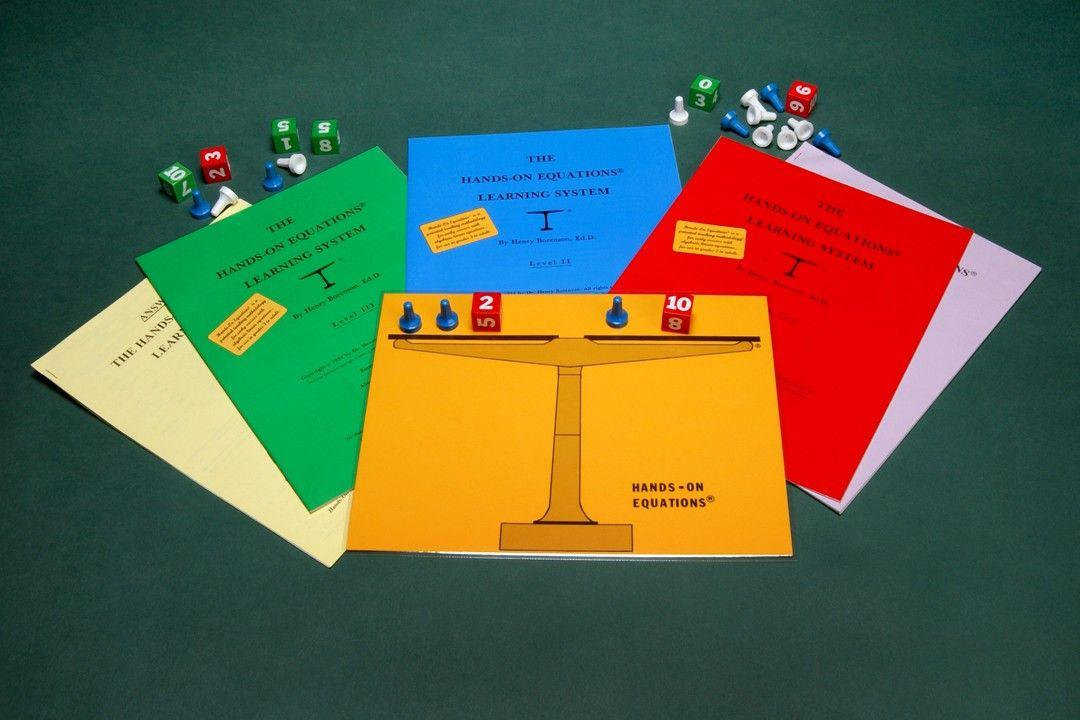# The Hand's-On Equations Learning System

### Making Algebra Child’s Play!The Hands-On Equations Learning System consists of three levels.  At each level, students use the game pieces to physically “set up” the given equation on the laminated balance scale. They then use “legal moves” to physically solve the equation. Students also learn to solve the equations using a pictorial solution.

#### Hands-On Equations is an innovative teaching methodology that was awarded a U.S. patent. It is a program that:

• Simplifies the teaching of algebraic concepts
• Makes algebraic concepts accessible to students in grades 3 – 8
• Uses the game pieces to physically represent and solve algebraic linear equations
• Enables students to enjoy their learning experience
• Promotes greater interest in mathematics
• Enhances student self-esteem
• Enables students to physically represent and solve word problems
• Provides students with a solid foundation for later algebraic learning
• Does not require any algebraic prerequisites

# Level II: Lessons 8 - 16 (Blue Booklet)

#### Students use the red cubes, blue pawns, and white pawns to set up and solve equations such as: 2x = star + 6 and 2x – star + 2 = star + 10.

##### Note: Star, written as x, is a new mathematical notation developed by Dr. Borenson for the opposite of x. It is a more compact symbol than the traditional notation, (-x). See note following the video below.

After learning the algebraic concepts using the star notation and the white pawn, students transfer their learning and make sense of examples such as 2x + (-x) + 3 = 2(-x) + 12 and 2x – (-x) = 15.